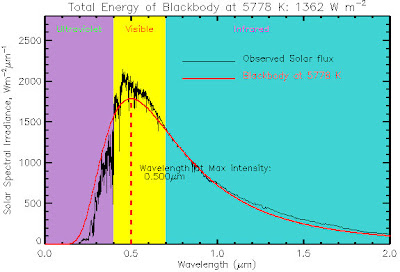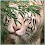## Sunday, August 15, 2010

### Is the 'Greenhouse Effect' based on a 'cool' Sun?

Most descriptions of the 'greenhouse effect' are similar to that offered by Wikipedia: "If an ideal thermally conductive blackbody was the same distance from the Sun as the Earth, it would have an expected blackbody temperature of 5.3°C. However, since the Earth reflects about 30%... of the incoming sunlight, the planet's actual blackbody temperature is about -18 or -19°C, about 33°C below the actual surface temperature of about 14 °C or 15 °C. The mechanism that produces this difference between the actual temperature and the blackbody temperature is due to the atmosphere and is known as the greenhouse effect."
This calculation is based upon the Stefan-Boltzmann law, radius of the Sun and Earth, distance between the Sun and Earth, and albedo (reflection, mostly from clouds), from which the following equation is derived:
where
Te = Temperature of the Earth surface
Ts = Temperature of the Sun surface
Rs = Radius of the Sun = 6.96x10^8 m
a   = Albedo (reflection of incoming solar radiation mostly from clouds) = 0.306
E  = IR emissivity of the Earth (assumed to be ~1)
D  = the Astronomical Unit = distance between the Sun and Earth = 1.496x10^11 m
If we plug in the value for the temperature of the Sun (5778°K) used by the Wikipedia article, we find that the temperature of the Earth without an atmosphere (setting albedo to 0) should be 278.68°K (5.53°C). This is very close to the 5.3°C ideal blackbody temperature as stated above. If we then set albedo to the commonly used value of 0.306 for Earth with an atmosphere, we find the Te calculation drops to -18.8°C. The 'greenhouse effect' is then calculated from the globally 'averaged' earth surface temperature of 15°C - -18.8 = 33.8°C. Note also the G&T paper has shown that it is not possible to calculate an 'average' Earth surface temperature (p.70-71), which also renders the 'greenhouse effect' calculation moot. In addition, all of these calculations are based on the incorrect assumption that the Earth, atmosphere, and Sun can each behave as ideal blackbodies.
The value for Sun surface temperature used in these calculations (5778°K or 5505°C) is a low estimate and thus biases the calculation to show an enhanced 'greenhouse effect.' The reason why is shown in the graph below of the observed distribution of solar radiation reaching the top of the atmosphere as a function of wavelength (solid black line).Ts based on wavelength at Max Intensity
We see that the wavelength at maximum intensity is in the visible spectrum at about .475 microns or 475 nanometers, which is what the Handbook of Chemistry and Physics, 82nd Edition, CRC Press, 2001 also shows as the maximum 'Solar Spectral Irradiance.' The Wein displacement law can then be used to calculate the Sun surface temperature based upon the maximum irradiance wavelength, resulting in a Sun surface temperature of 6101°K (5828°C). Another source gives an even higher figure, "The Sun's outer visible layer is called the photosphere and has a temperature of 6000°C," which is equivalent to 6273°K.
If we had used the Sun surface temperature of 5778°K assumed by the Wikipedia article, we would find that the Sun emitted more energy than an ideal blackbody throughout the visible spectrum, which is impossible:Solar flux exceeds blackbody Wikipedia Ts ,
If we now use the Sun surface temperature calculated from Wein's displacement law as the input to the equation above, we find the Earth surface temperature without an atmosphere should be 294.26°K or 21.11°C. Since the 'average' Earth surface temperature is commonly cited as 15°C, that means the Earth with a 'greenhouse gas' atmosphere along with the additional factors of convection, evapo-transpiration, and adiabatic effects is colder than an Earth without!
see also the peer-reviewed Chilingar et al paper Cooling of the atmosphere due to CO2
h/t comments by Gord, graph source

1.Is it possible to work anything out regarding the correct average Sun temperature by looking at temperature data from the moon, which obviously does have no atmosphere?

2.Let's plug it in.
The albedo of the Moon is 0.12. In other words, the Moon reflects back 12% of all the radiation that falls upon it - from the Universe Today website.

In the day, the temperature of the Moon averages 107 C, although it rises as high as 123 C. The night cools the surface to an average of -153 C, or -233 C in the permanently shaded south polar basin. A typical non-polar minimum temperature is -181 C (at the Apollo 15 site).
The Lunar temperature increases about 280 C from just before dawn to Lunar noon. Average temperature also changes about 6 C betwen aphelion and perihelion - from the Artemis Project website.

Let's take the typical situation. average 107 C and -181 C = -74 C. + the average of the aphelion versus perihelion temp change of 6. (Cut it in half?)

Tm = -71 C.
Ts = same as above
Rs = same
am = (Albedo of the Moon) = 0.12

E = IR emissivity of the Moon (can we assume to be ~1 also?)
D = the Astronomical Unit = distance between the Sun and Moon = 1.496x10^11 m = (compensated for with the aphelion/perihelion temp difference)

From this point on I am mathematicly challenged, and don't mind handing the problem to the next in line.
Good luck ;)

Then again there are better measurements being taken on a daily basis. The info given isn't complete enough for our purpose however.
I'd stick with the other - see how it turns out.

3.All the "blackbody" calculations are not applicable to the real world, anyway, because real objects "soak in" heat and don't emit all they absorb.

4.jae, yes...the post states the Sun, atmosphere, and Earth are not ideal blackbodies and therefore not ideal absorbers or emitters.

5.MS, yes, yes, but I brought this up because I think the post is looking more at the effects of absorptivity and emissivity, not heat storage.

6.The Moon average temp minus -71°C.

The Earth without greenhouse effect expected blackbody temperature of 5.3°C, in other words the temp without an atmosphere.

Why is the "airless" Earth warmer then the moon?

7.papertiger,

a moon day lasts 29.5 Earth days and thus has much longer time to cool off than an Earth day, and much longer time to heat up as well. This involves the fallacy of trying to compute an 'average' temperature as noted by G&T. Apparently not possible to do and thus another reason why calculations of 'greenhouse effects' are moot.

8.papertiger,
Also meant to say the cooling curve is different from the heating curve. The cooling curve shows temperatures decrease at an exponentially decreasing rate while (I think) the heating curve is more linear until equilibrium. Thus, the length of day can make a big difference between the 2 planets.

9.What's the energy input of the work done by the gravity of the moon as tidal motion?

10.These simple calculation don't take into account the insulating properties of the atmosphere, which has R value of about 0.85, a value I found by a google search.

I wonder if an engineer whose speciality is heat transfer and insulation has done the calculations of the earth's temperature using the atmosphere's R value.

Harold Pierce Jr

11.Hockey Schtick,

This equation relates Sun Temperature to Earth Temperature:

TE = TS (((1-a)^0.5 * Rs)/(2*D)))^0.5)
Where TE is blackbody temp of the Earth in K
TS is the surface temp of the SUN in K
Rs is radius of the Sun (6.96 X 10^8 meters)
D is distance between the Sun and Earth in meters (1.5 X 10^11)
a is albedo of the Earth

The equation you posted on your website is not correct.
TE = TS ((((1-a)/e)^0.5 * Rs)/(2*D)))^0.5)

This equation has e = emissivity of the Earth included again.
(1-a) is equal to e and it already includes the emissivity of the Earth as well as the Clouds.

Division by e is obviously wrong since if e = 0 (a perfect reflecting Earth), it will produce an INFINITE Earth temperature (TE)!

Someone has changed the correct equation on Wikipedia to this hilarious and obviously wrong form.

Gord.

12.Here is a good explaination of the Moon's temperature.

A Greenhouse Effect on the Moon?

"As the chart and the study indicate, actual daytime lunar temperatures were lower than expected because the real moon also conducts heat to the inside rather than radiating all of it to space. Conversely, actual surface temperatures throughout its two-week night were higher than expected because the moon "feeds on" the heat it had previously absorbed. Thus (within the zone in question) the surface of the real moon is
roughly 20° cooler than predicted by day and 60° warmer by night, the net result being a surface that is 40° warmer than predicted.

To quote NASA's analysis,
During lunar day, the lunar regolith absorbs the radiation from the sun and transports it inward and is stored in a layer approximately 50cm thick. As the moon passes into night, the radiation from the sun quickly approaches zero (there is still a bit of radiation from the earth) and, in contrast with a precipitous drop in temperature if it was a simple black body, the regolith then proceeds to transport the stored heat back onto the surface, thus warming it up significantly over the black body approximation.
All without greenhouse gases."

Conclusion
"The Earth is not “unusually” warm. It is the application of the predictive equation that is faulty. The ability of common substances to store heat makes a mockery of blackbody estimates. The belief that radiating
trace gases explain why earth's surface temperature deviates from a simple mathematical formula is based
on deeply erroneous assumptions about theoretical vs. real bodies. These faulty assumptions are discussed
in detail elsewhere, where they are shown to lead to the 'cold earth fallacy' ."

Martin Hertzberg, PhD, Consultant in Science and Technology
Hans Schreuder, retired analytical chemist
13.